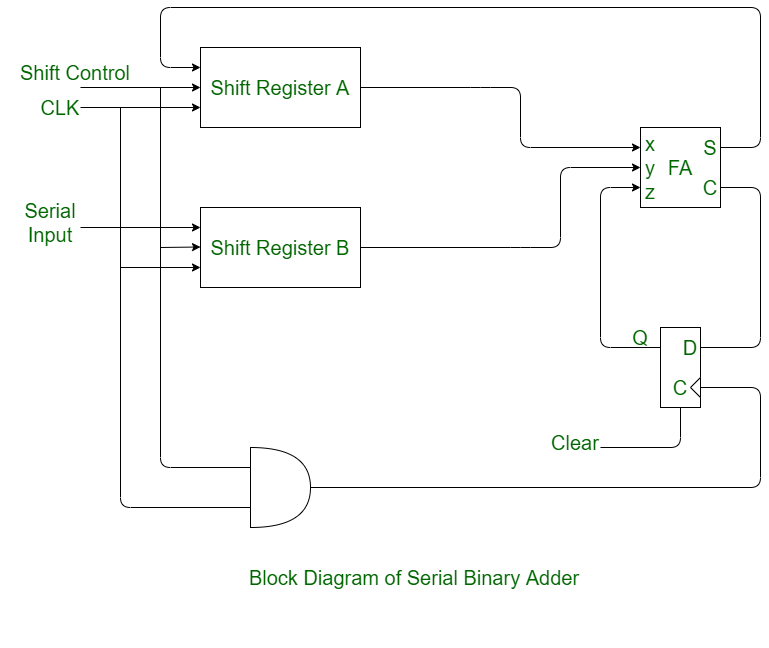# Serial Binary Adder in Digital Logic

• Difficulty Level : Basic
• Last Updated : 22 Apr, 2020

Serial binary adder is a combinational logic circuit that performs the addition of two binary numbers in serial form. Serial binary adder performs bit by bit addition. Two shift registers are used to store the binary numbers that are to be added.

A single full adder is used to add one pair of bits at a time along with the carry. The carry output from the full adder is applied to a D flip-flop. After that output is used as carry for next significant bits. The sum bit from the output of the full adder can be transferred into a third shift register.

Block diagram of Serial Binary Adder:Shift Registers :
Shift Register is a group of flip flops used to store multiple bits of data. There are two shift registers used in the serial binary adder. In one shift register augend is stored and in other shift register addend is stored.

Full adder is the combinational circuit which takes three inputs and gives two outputs as sum and carry. The circuit adds one pair at a time with the help of it.

D Flip-flop :
the carry output from the full adder is applied on the D flip-flop. Further, the output of D flip-flop is used as a carry input for the next pair of significant bits.

Working Process:

• Step-1:
The two shift registers A and B are used to store the numbers to be added.
• Step-2:
A single full adder is used too add one pair of bits at a time along with the carry.
• Step-3:
The contents of the shift registers shift from left to right and their output starting from a and b are fed into a single full adder along with the output of the carry flip-flop upon application of each clock pulse.
• Step-4:
The sum output of the full adder is fed to the most significant bit of the sum register.
• Step-5:
The content of sum register is also shifted to right when clock pulse is applied.
• Step-6:
After applying four clock pulse the addition of two registers (A & B) contents are stored in sum register.
My Personal Notes arrow_drop_up